# 从零开始的深度学习 Ch1

## Perceptron & Neural Network

Posted by R1NG on January 8, 2021 Viewed Times

# 感知机与神经网络

## 1. 感知机的定义

1. 输入 (Input)
2. 与每一个输入所对应的权值 (Weights)
3. 激活函数 (Function)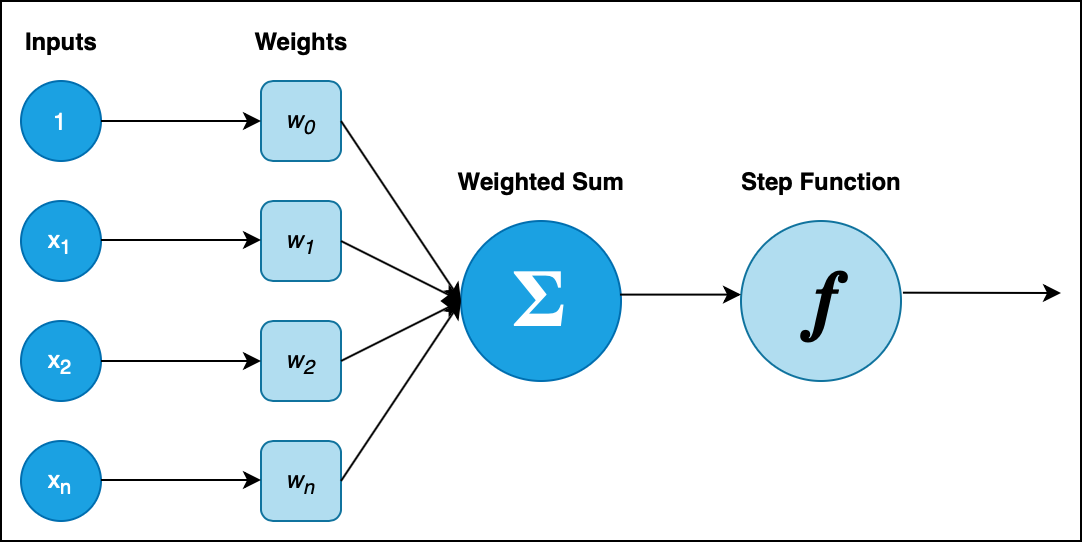$X = (1, x_1, \cdots, x_n),~~ W = (w_0, w_1, \cdots, w_n).$

$f(X) = \begin{cases} 0 ~~~~~~ X \cdot W^T \leqslant \theta \\ 1 ~~~~~~ X \cdot W^T > \theta \end{cases}$

[例] 使用感知机实现与, 或, 与非门:

$x_1$ $x_2$ AND OR NAND
$1$ $1$ $1$ $1$ $0$
$1$ $0$ $0$ $1$ $1$
$0$ $1$ $0$ $1$ $1$
$0$ $0$ $0$ $0$ $1$
1
2
3
4
5
6
7
8
9
10
11
12
13
14
15
16
17
18
19
20
21
22
23
24
25
26
27
28
29
30
31
32
import numpy as np

def AND(x1, x2):
x = np.array([x1, x2])
w = np.array([0.5, 0.5])
b = -0.7
tmp = np.sum(w*x) + b
if tmp <= 0:
return 0
else:
return 1

def OR(x1, x2):
x = np.array([x1, x2])
w = np.array([0.5, 0.5])
b = -0.2
tmp = np.sum(w*x) + b
if tmp <= 0:
return 0
else:
return 1

def NAND(x1, x2):
x = np.array([x1, x2])
w = np.array([-0.5, -0.5])
b = 0.7
tmp = np.sum(w*x) + b
if tmp <= 0:
return 0
else:
return 1


## 2. 感知机的局限性和扩展的多层感知机: 以异或门电路的实现为例

$x_1$ $x_2$ XOR
$1$ $1$ $0$
$1$ $0$ $1$
$0$ $1$ $1$
$0$ $0$ $0$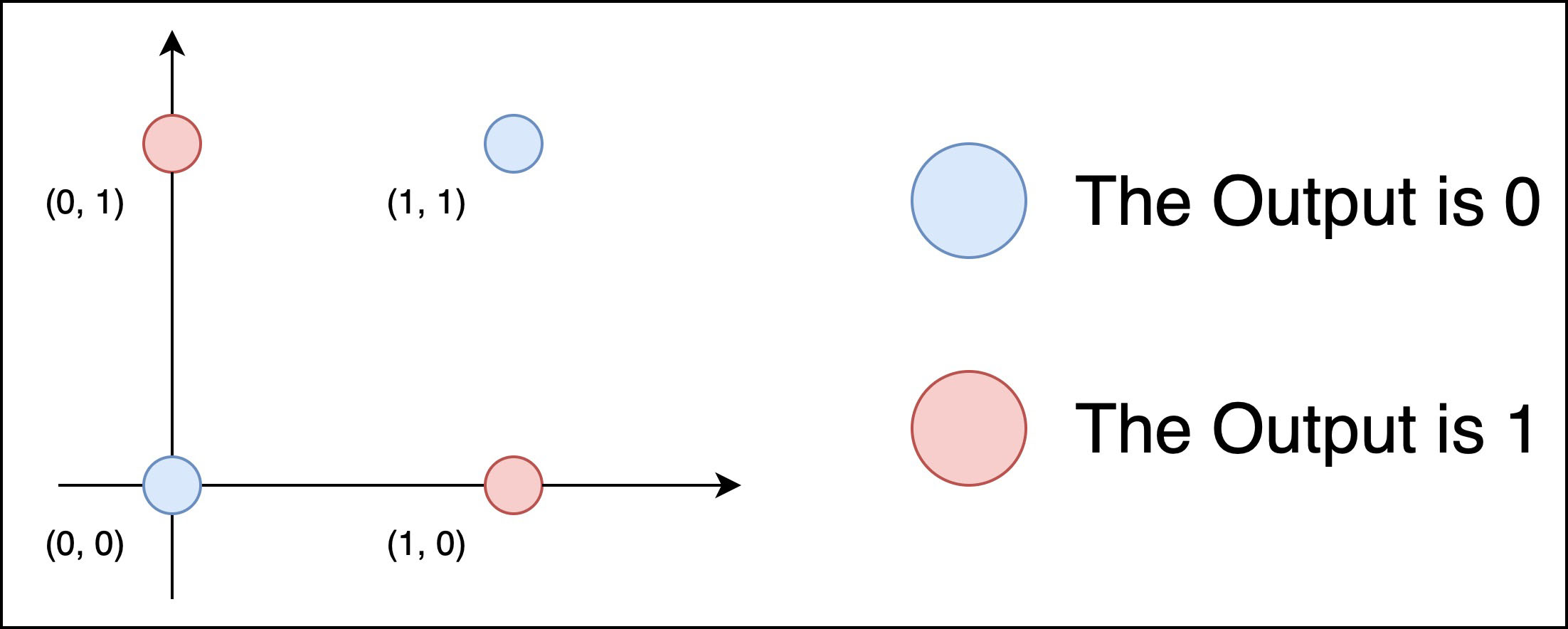$\mathbf{XOR}(x_1, x_2) = \mathbf{AND} (\mathbf{NAND}(x_1, x_2), \mathbf{OR}(x_1, x_2))$

1
2
3
4
5
def XOR(x1, x2):
s1 = NAND(x1, x2)
s2 = OR(x1, x2)
y = AND(s1, s2)
return y


## 3. 神经网络的定义和层级结构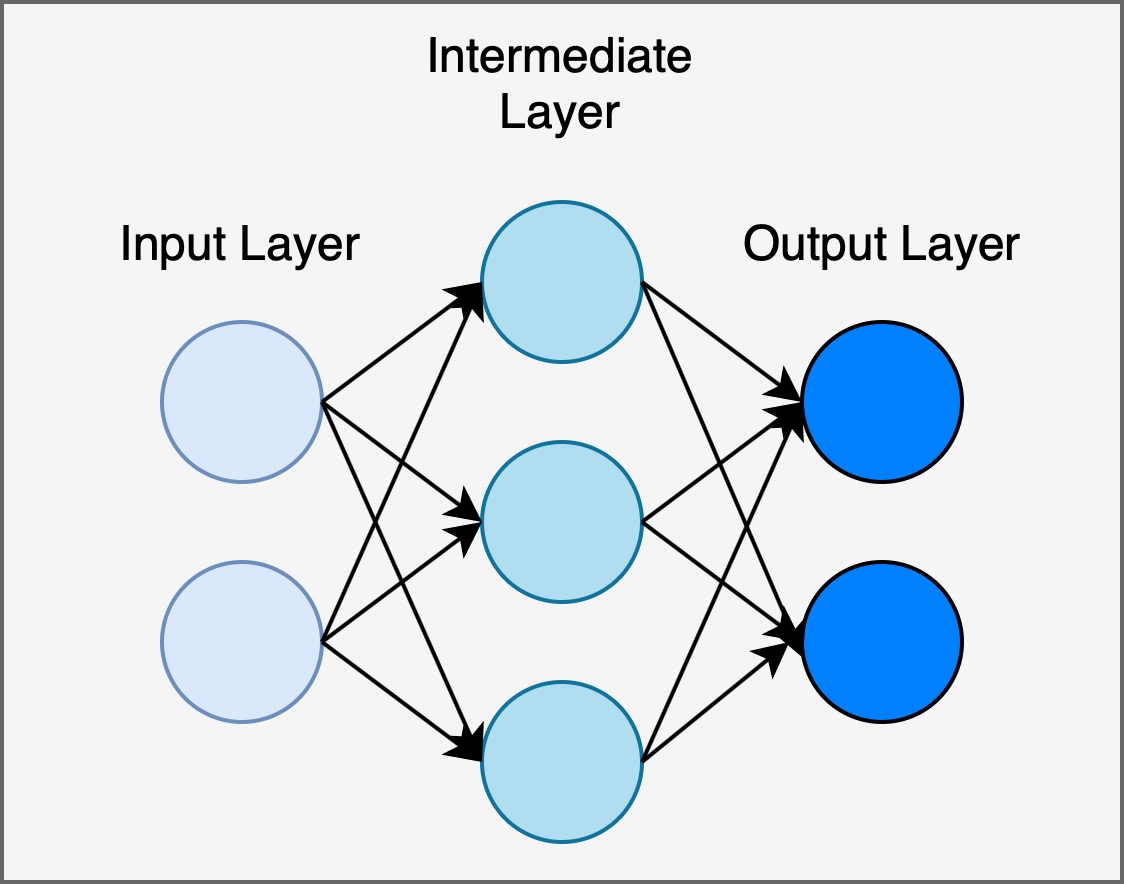## 4. 激活函数的定义和常见的激活函数

1. Sigmoid 函数
Sigmoid 函数 $S(x)$:

$S(X) = \frac{1}{1 + \exp^{(-x)}}.$

Python 中, Sigmoid 函数实现如下:

1
2
3
4
import numpy as np

def sigmoid(X):
return 1/ (1 + np.exp(-x))


2. ReLU 函数
ReLU 函数 $r(x)$:

$r(X) = \begin{cases} x ~~~ (x > 0) \\ 0 ~~~ (x \leqslant 0)\end{cases}$

Python 中, ReLU 函数实现如下:

1
2
3
4
import numpy as np

def relu(X):
return np.maximum(0, x)


1. softmax 函数
softmax 函数 $s(x)$:

$S(x) = \frac{\exp(a_k)}{\sum_{1}^{n}exp(a_i)}$

需要注意的是, softmax 函数的实现中涉及指数函数计算, 而在指数函数值过大时可能会溢出为 inf. 若分子和分母均溢出的话, 就无法正常地进行除法运算.

要解决这一问题, 我们对 softmax 函数作如下修正:

$m = \max(a_1, a_2, \cdots, a_n)$ $S_1(x) = \frac{\exp(a_k - m)}{\sum_{1}^{n}exp(a_i - m)}$

这样, 就在不改变运算的结果 (思考一下: 为什么?) 的情况下, 实现了函数的修正. 合理的 Python 实现如下:

1
2
3
4
5
6
7
8
9
import numpy as np

def softmax(a):
c = np.max(a)
exp_a = np.exp(a - c)
sum_exp_a = np.sum(exp_a)
y = exp_a / sum_exp_a

return y


softmax 函数的一个有趣的特性是, 对任何输入值, 其函数值均在 $0, 1$ 之间, 且输出总和为 $1$. 基于这个性质, 我们可以将函数的输出解读为概率, 并用概率的工具和方法处理问题.

## 5. 三层神经网络的实现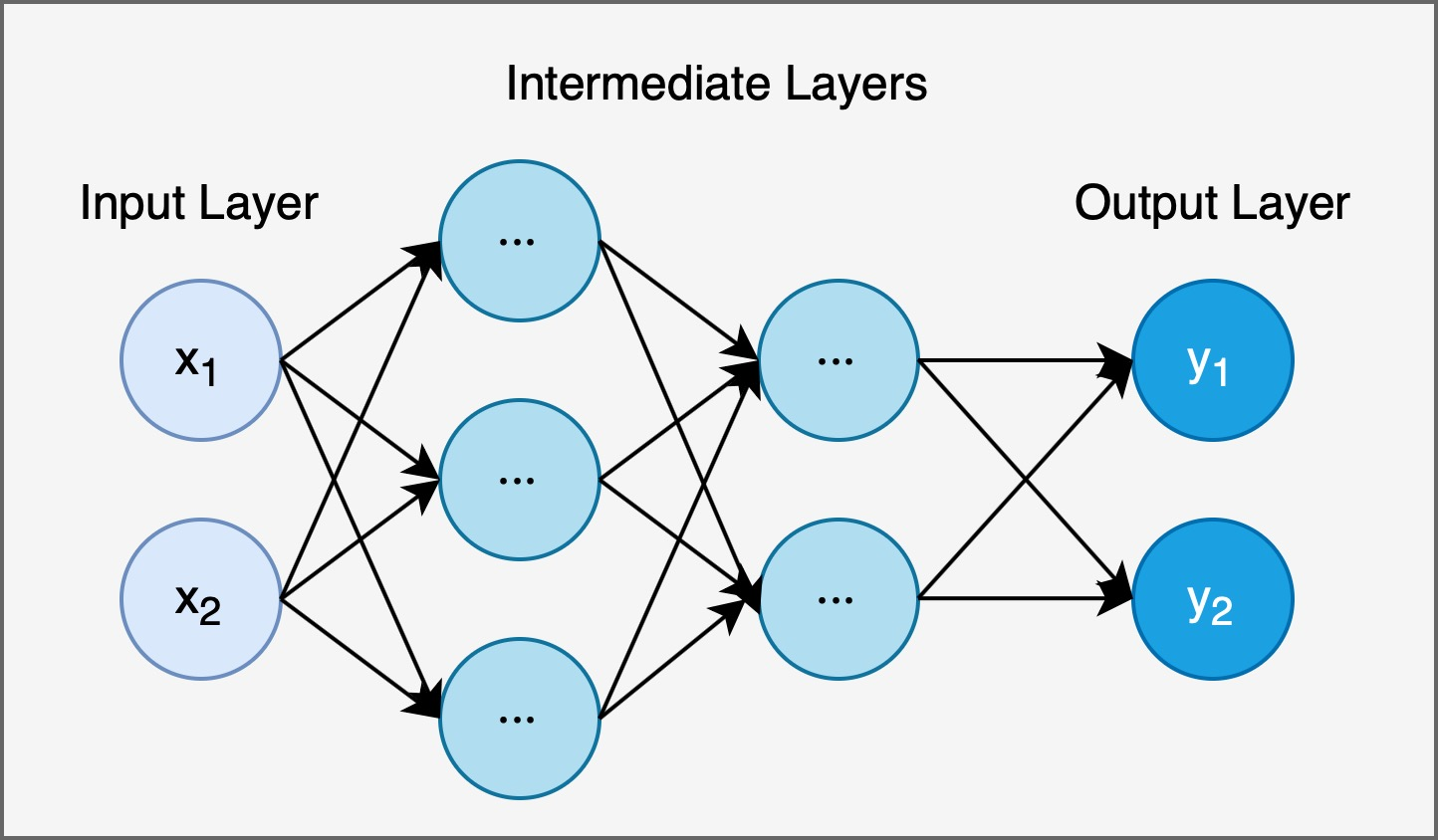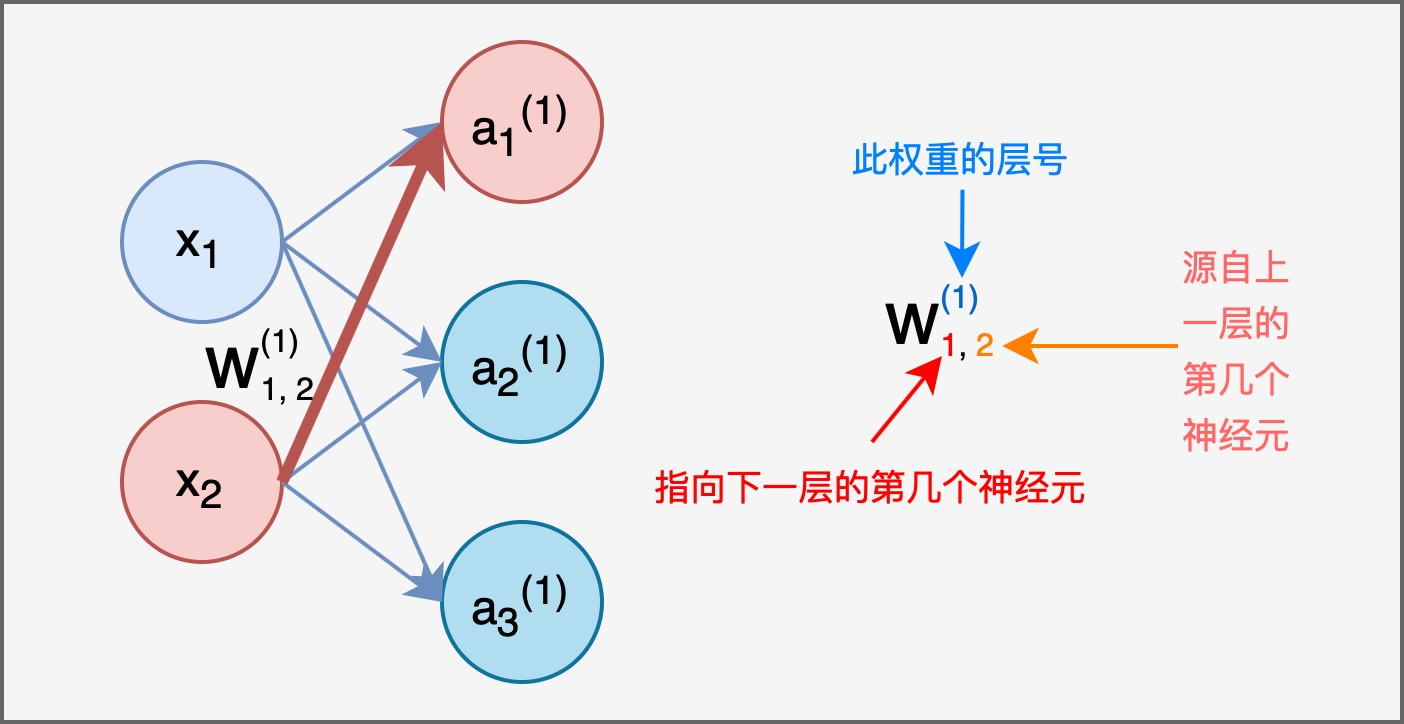$a_{1}^{(1)} = w_{11}^{(1)}x_1 + w_{12}^{(1)}x_2 + b_1$

$A^{(1)} = (a_{1}^{(1)}, a_{2}^{(1)}, a_{3}^{(1)}), ~~~ X = (x_1, x_2), ~~~ B^{(1)} = (b_{1}^{(1)}, b_{2}^{(1)}, b_{1}^{(3)})$ $W^{(1)} = \begin{pmatrix} w^{(1)}_{11}, w^{(1)}_{21}, w^{(1)}_{31} \\ ~ \\ w^{(1)}_{12}, w^{(1)}_{22}, w^{(1)}_{32} \end{pmatrix}$ $A^{(1)} = XW^{(1)} + B^{(1)}$

1
2
3
4
5
6
7
8
9
10
11
12
13
14
15
16
17
18
19
20
21
22
23
24
25
26
27
28
29
30
31
from functions import sigmoid, identity_function
import numpy as np

def init_network():
network = {}
network['W1'] = np.array([[0.1, 0.3, 0.5], [0.2, 0.4, 0.6]])
network['b1'] = np.array([0.1, 0.2, 0.3)
network['W2'] = np.array([[0.1, 0.4], [0.2, 0.5], [0.3, 0.6]])
network['b2'] = np.array([0.1, 0.2)
network['W3'] = np.array([[0.1, 0.3] ], [0.2, 0.4]])
network['b3'] = np.array([0.1, 0.2)

return network

def forward(network, x):
W1, W2, W3 = network['W1'], network['W2'], network['W3']
b1, b2, b3 = network['b1'], network['b2'], network['b3']

a1 = np.dot(x, W1) + b1
z1 = sigmoid(a1)
a2 = np.dot(z1, W2) + b2
z2 = sigmoid(a2)
a3 = np.dot(z2, W3) + b3
y = identity_function(a3)

return y

network = init_network()
x = np.array([1.0, 0.5])
y = forward(network, x)
print(y)    #the output should be [0.31682708 0.69627909]


## 6. 神经网络的输出

MNIST 的图像数据为 $28$px * $28$px 的灰度图像. 依照图片所包含的像素数量和我们需要识别的数字种类, 确定神经网络的输入层有 $784$ 个神经元, 输出层有 $10$ 个神经元. 其隐藏层又由 $50$ 个神经元构成的第一隐藏层和 $100$ 个神经元构成的第二隐藏层组成. 在原书提供的源代码中, 提供了现成的 MNIST 数据集抓取和转换函数, 而神经网络的权值保存在 sample_weight.pkl 这个 Pickel 文件中, 在定义神经网络时被直接读取.

1
2
3
4
5
6
7
8
9
10
11
12
13
14
15
16
17
18
19
20
21
22
23
24
25
26
27
28
29
30
31
32
33
34
35
36
37
38
39
40
41
42
43
44
45
import sys, os
sys.path.append(os.pardir)  # 为了导入父目录的文件而进行的设定

import numpy as np
import pickle
from common.functions import sigmoid, softmax

def get_data():
(x_train, t_train), (x_test, t_test) = load_mnist(normalize=True, flatten=True, one_hot_label=False)
return x_test, t_test

def init_network():
with open("sample_weight.pkl", 'rb') as f:
return network

def predict(network, x):
W1, W2, W3 = network['W1'], network['W2'], network['W3']
b1, b2, b3 = network['b1'], network['b2'], network['b3']

a1 = np.dot(x, W1) + b1
z1 = sigmoid(a1)
a2 = np.dot(z1, W2) + b2
z2 = sigmoid(a2)
a3 = np.dot(z2, W3) + b3
y = softmax(a3)

return y

x, t = get_data()
network = init_network()
accuracy_cnt = 0
for i in range(len(x)):
y = predict(network, x[i])
p= np.argmax(y) # 获取概率最高的元素的索引

if p == t[i]:
accuracy_cnt += 1

print("Accuracy:" + str(float(accuracy_cnt) / len(x)))


1
Accuracy:0.9352